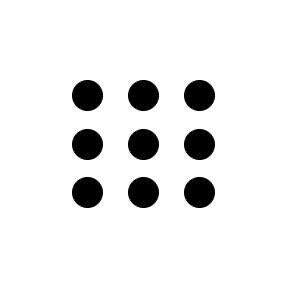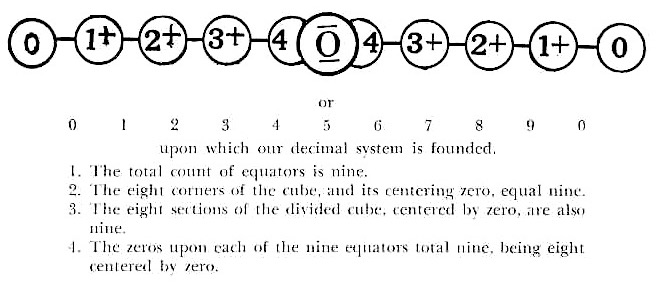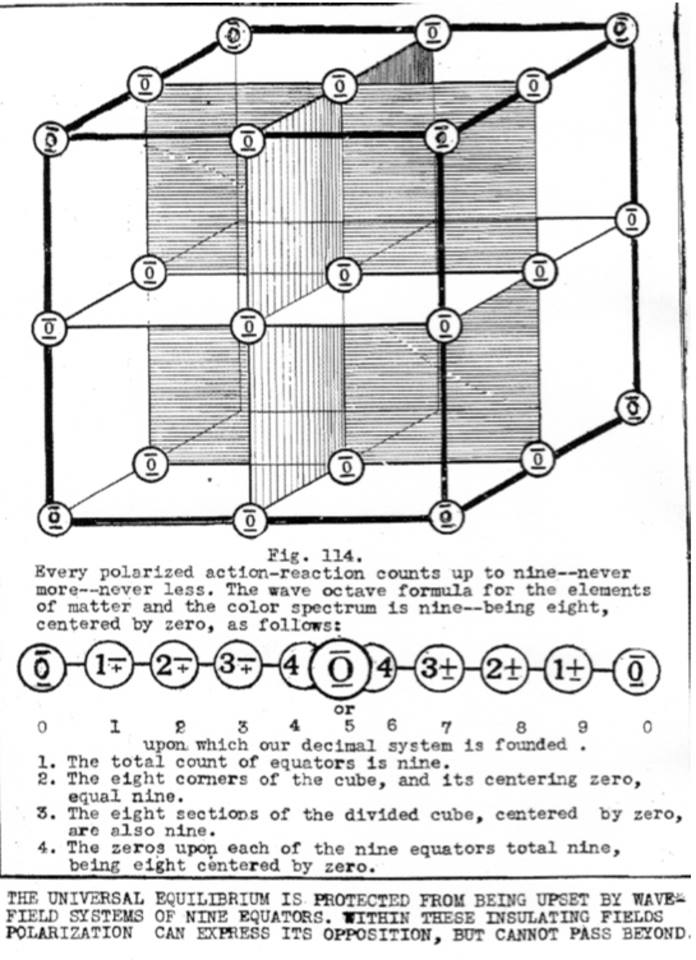# Nine

 NineNine

noun: the cardinal number that is the sum of eight and one, three squared. adjective: denoting a quantity consisting of one more than eight and one less than ten

"And all that are three are nine - for all that are three are multiplied by three in this visible cube dominated universe of three dimensions." [Atomic Suicide, page 109]

"Every polarized action-reaction counts up to nine – never more – never less. The wave octave formula for the elements of matter and the color spectrum is nine – being eight, centered by zero, as follows:(courtesy University of Science and Philosophy) (click to enlarge)

upon which our decimal system is founded. 1. The total count of equators is nine. 2. The eight corners of the cube, and its centering zero, equal nine. 3. The eight sections of the divided cube, centered by zero, are also nine.

4. The zeros upon each of the nine equators total nine, being eight centered by zero. [Atomic Suicide, page 113](courtesy University of Science and Philosophy) (click to enlarge)